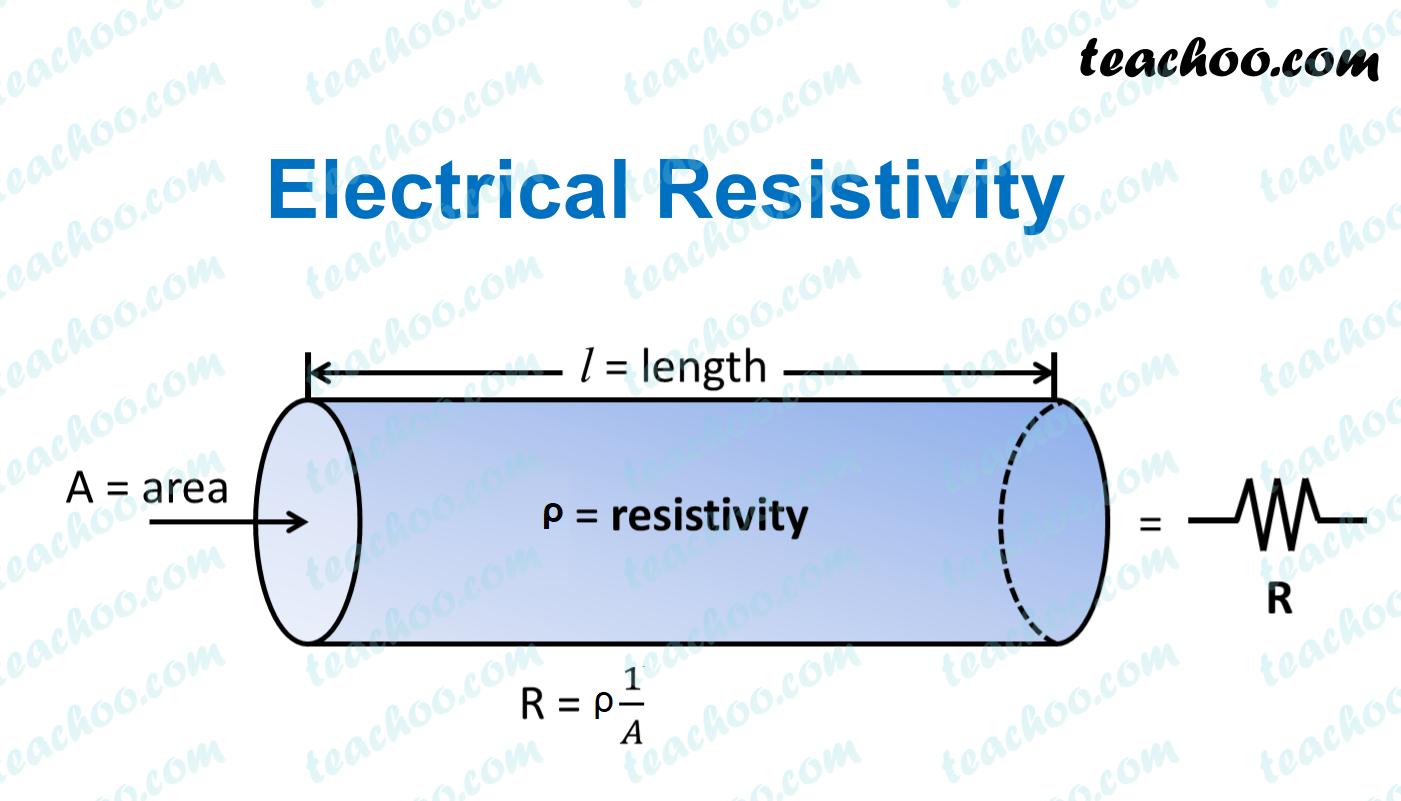Past Year - 1 Mark Questions

Class 10
Chapter 12 Class 10 - Electricity#### SI Unit of resistivity is ohm meter.

Explanation.

We know that,

R = ρ (l/A)

Therefore,

ρ = R (A/l)

= ohm × m 2 /m

= ohm × m

Therefore, unit of rho is ohm meter.

Note: Unit of R is ohm, Unit of area of cross-section is m 2 , unit of length is m

Learn in your speed, with individual attention - Teachoo Maths 1-on-1 Class